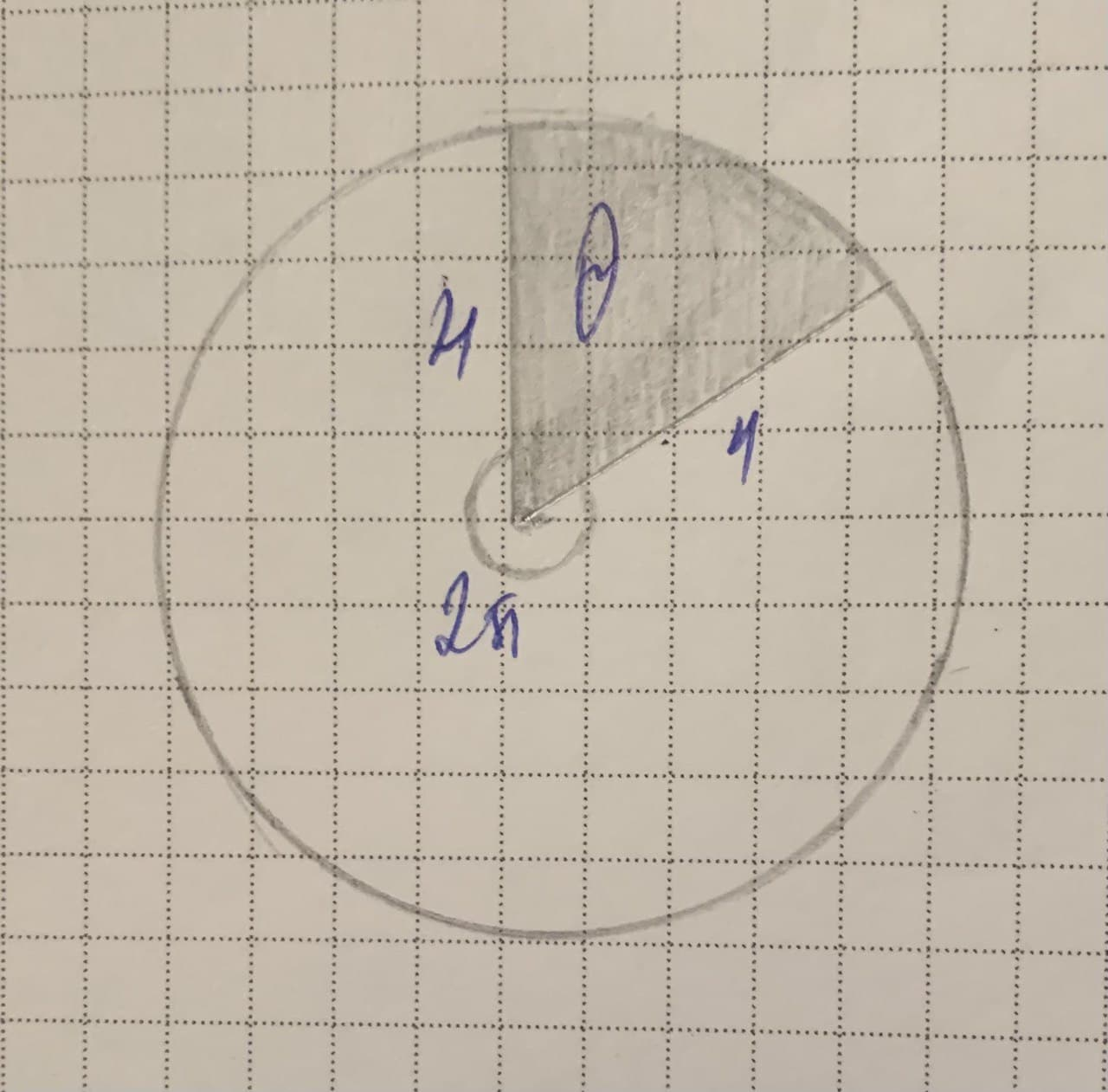# The minute hand of a certain clock is 4 inWanda Kane 2021-12-18 Answered
The minute hand of a certain clock is 4 in long, Starting from the moment when the hand is pointing straight up, how fast is the area of the sector that is swept out by the hand increasing at any instant during the next revolution of the hand?
You can still ask an expert for help

• Live experts 24/7
• Questions are typically answered in as fast as 30 minutes
• Personalized clear answers

Solve your problem for the price of one coffee

• Math expert for every subject
• Pay only if we can solve ityotaniwc

We know that : (see the picture)
$\therefore A=\frac{1}{2}{r}^{2}\theta$
$\frac{dA}{dt}=\frac{1}{2}{r}^{2}\frac{d\theta }{dt}$ (r is constant)
The minute hand takes 60 minutes for one revolution. Then the angular velocity of one revolution per minute is
$\frac{d\theta }{dt}=\frac{2\pi }{60}=\frac{\pi }{30}\frac{rad}{min}$
Thus,
$\therefore \frac{dA}{dt}=\frac{4\pi }{15}\frac{{\in }^{2}}{min}$We have step-by-step solutions for your answer!raefx88y
1. Speed of the minute hand is constant = 360 degrees per hour, or $2\pi \frac{radians}{hr}$
2. Sector area $=\frac{\theta }{2\pi }\pi {r}^{2}{\in }^{2}=\frac{1}{2}{r}^{2}\theta {\in }^{2}=\frac{16}{2}\theta {\in }^{2}=8\theta {\in }^{2}$
3.$\frac{d\theta }{dt}=\frac{2\pi }{60}\frac{radians}{min}$
4. $\frac{d}{dt}$ (sector area) $=\frac{d}{dt}8\theta =8\frac{d}{dt}\theta =\frac{16\pi }{60}=\frac{4}{15}\pi \frac{{\in }^{2}}{min}$

We have step-by-step solutions for your answer!